Publicité

# Pptgravity 091010165847-phpapp01

30 Aug 2015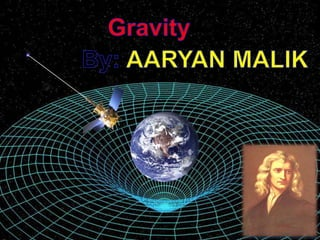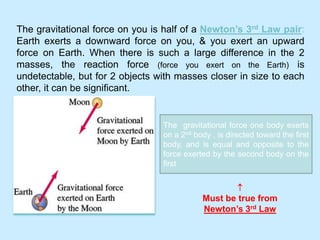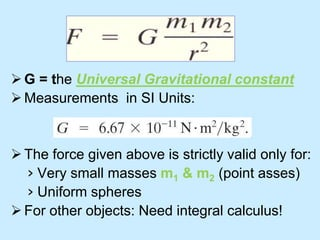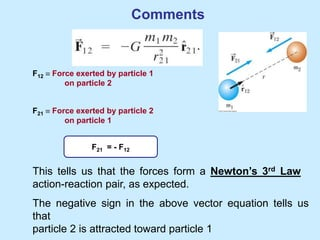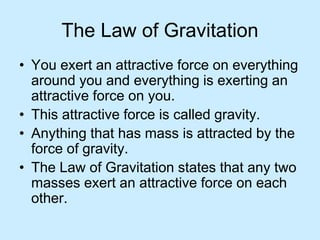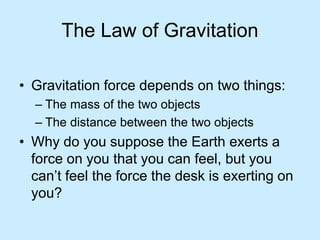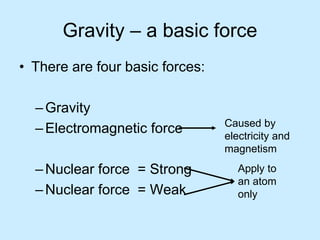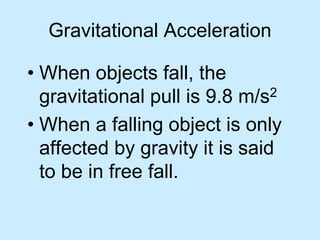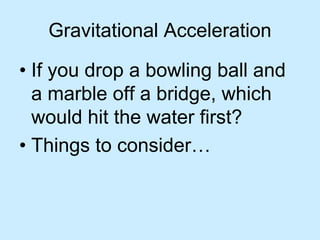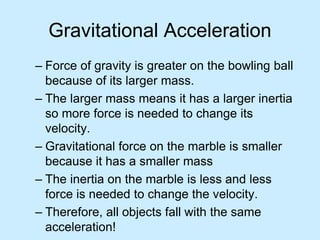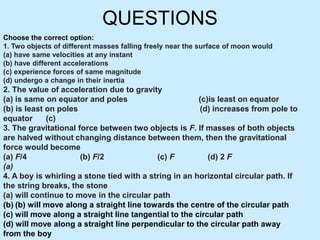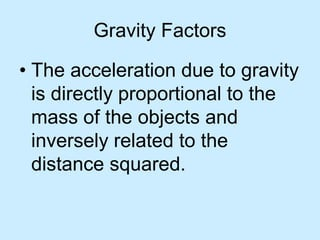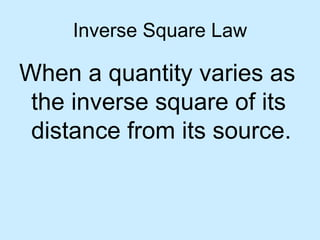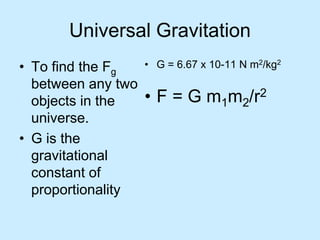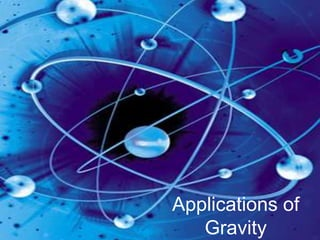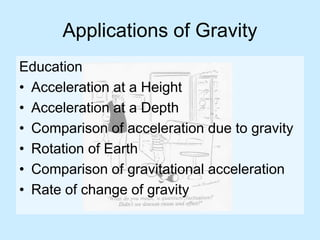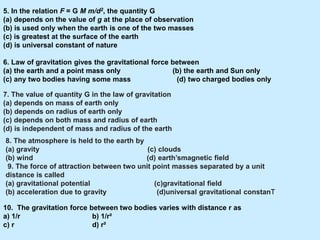1 sur 18
Publicité

### Pptgravity 091010165847-phpapp01

1. GRAVITY
2.  Must be true from Newton’s 3rd Law The gravitational force on you is half of a Newton’s 3rd Law pair: Earth exerts a downward force on you, & you exert an upward force on Earth. When there is such a large difference in the 2 masses, the reaction force (force you exert on the Earth) is undetectable, but for 2 objects with masses closer in size to each other, it can be significant. The gravitational force one body exerts on a 2nd body , is directed toward the first body, and is equal and opposite to the force exerted by the second body on the first
3. G = the Universal Gravitational constant Measurements in SI Units: The force given above is strictly valid only for: › Very small masses m1 & m2 (point asses) › Uniform spheres For other objects: Need integral calculus!
4. Comments F12  Force exerted by particle 1 on particle 2 F21  Force exerted by particle 2 on particle 1 This tells us that the forces form a Newton’s 3rd Law action-reaction pair, as expected. The negative sign in the above vector equation tells us that particle 2 is attracted toward particle 1 F21 = - F12
5. The Law of Gravitation • You exert an attractive force on everything around you and everything is exerting an attractive force on you. • This attractive force is called gravity. • Anything that has mass is attracted by the force of gravity. • The Law of Gravitation states that any two masses exert an attractive force on each other.
6. The Law of Gravitation • Gravitation force depends on two things: – The mass of the two objects – The distance between the two objects • Why do you suppose the Earth exerts a force on you that you can feel, but you can’t feel the force the desk is exerting on you?
7. Gravity – a basic force • There are four basic forces: –Gravity –Electromagnetic force –Nuclear force = Strong –Nuclear force = Weak Apply to an atom only Caused by electricity and magnetism
8. Gravitational Acceleration • When objects fall, the gravitational pull is 9.8 m/s2 • When a falling object is only affected by gravity it is said to be in free fall.
9. Gravitational Acceleration • If you drop a bowling ball and a marble off a bridge, which would hit the water first? • Things to consider…
10. Gravitational Acceleration – Force of gravity is greater on the bowling ball because of its larger mass. – The larger mass means it has a larger inertia so more force is needed to change its velocity. – Gravitational force on the marble is smaller because it has a smaller mass – The inertia on the marble is less and less force is needed to change the velocity. – Therefore, all objects fall with the same acceleration!
11. QUESTIONS Choose the correct option: 1. Two objects of different masses falling freely near the surface of moon would (a) have same velocities at any instant (b) have different accelerations (c) experience forces of same magnitude (d) undergo a change in their inertia 2. The value of acceleration due to gravity (a) is same on equator and poles (c)is least on equator (b) is least on poles (d) increases from pole to equator (c) 3. The gravitational force between two objects is F. If masses of both objects are halved without changing distance between them, then the gravitational force would become (a) F/4 (b) F/2 (c) F (d) 2 F (a) 4. A boy is whirling a stone tied with a string in an horizontal circular path. If the string breaks, the stone (a) will continue to move in the circular path (b) (b) will move along a straight line towards the centre of the circular path (c) will move along a straight line tangential to the circular path (d) will move along a straight line perpendicular to the circular path away from the boy
12. Gravity Factors • The acceleration due to gravity is directly proportional to the mass of the objects and inversely related to the distance squared.
13. Inverse Square Law When a quantity varies as the inverse square of its distance from its source.
14. Universal Gravitation • To find the Fg between any two objects in the universe. • G is the gravitational constant of proportionality • G = 6.67 x 10-11 N m2/kg2 • F = G m1m2/r2
15. Applications of Gravity
16. Applications of Gravity Education • Acceleration at a Height • Acceleration at a Depth • Comparison of acceleration due to gravity • Rotation of Earth • Comparison of gravitational acceleration • Rate of change of gravity
17. 5. In the relation F = G M m/d2, the quantity G (a) depends on the value of g at the place of observation (b) is used only when the earth is one of the two masses (c) is greatest at the surface of the earth (d) is universal constant of nature 6. Law of gravitation gives the gravitational force between (a) the earth and a point mass only (b) the earth and Sun only (c) any two bodies having some mass (d) two charged bodies only 7. The value of quantity G in the law of gravitation (a) depends on mass of earth only (b) depends on radius of earth only (c) depends on both mass and radius of earth (d) is independent of mass and radius of the earth 8. The atmosphere is held to the earth by (a) gravity (c) clouds (b) wind (d) earth’smagnetic field 9. The force of attraction between two unit point masses separated by a unit distance is called (a) gravitational potential (c)gravitational field (b) acceleration due to gravity (d)universal gravitational constanT 10. The gravitation force between two bodies varies with distance r as a) 1/r b) 1/r² c) r d) r²
18. The End for more:Log on to aaryanmalik/slidesh are.com THANK YOU!
Publicité